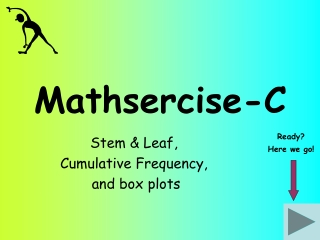DownloadDownload PresentationMathsercise-C

# Mathsercise-C

Télécharger la présentation## Mathsercise-C

- - - - - - - - - - - - - - - - - - - - - - - - - - - E N D - - - - - - - - - - - - - - - - - - - - - - - - - - -
##### Presentation Transcript

1. Mathsercise-C Stem & Leaf, Cumulative Frequency, and box plots Ready? Here we go!

2. 2 3 4 Complete this stem and leaf diagram for the weights of 10 newly born boys (in kg) 1 6 5 9 8 2 6 8 7 3.6 5 4.1 4.5 2.9 3.8 3.2 3.6 2.8 3.7 2.5 Question 2 Key: 4|1 = 4.1kg 1 Stem & Leaf Answer

3. The stem and leaf table shows the number of students late each day to school last month The median is the middle value! Question 3 Key: 3|0 = 30 Students 1 12 2 3 3 6 6 8 9 The range is the highest value subtract lowest value 0 1 5 2 1 6 9 3 4 6 0 0 2 2 2 37 7 Answer a) Find the median b) Work out the range 2 Cumulative Frequency - = 25

4. Question 4 55 50 45 40 35 30 25 20 15 10 5 2 4 6 8 12 14 16 10 18 60 students took a test. The graph shows information about their marks 60 a) What was the median mark? b) What was the lowest mark? Cumulative Frequency c) Estimate how many students scored 12 or less marks 20 d) Estimate the interquartile range = 4.5 15.5 - 14 5 11 20 3 Cumulative Frequency Marks Answer

5. 30 32 34 36 42 50 22 24 26 28 38 40 44 46 48 52 54 This box plot shows information about 40 students’ test marks Start Which of these statements are true? a) The top mark was 54 False - 53 b) The range was 35 False - 30 c) The median was 42 False - 38 True d) The interquartile range was 12 5 e) ½ the students scored less than 38 True 4 Cumulative Frequency Answer